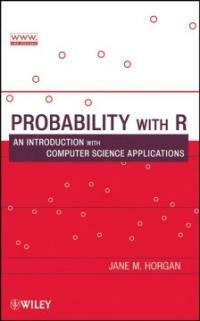> Detail View

# Detail View## Probability with R : an introduction with computer science applications

Material type
단행본
Personal Author
Horgan, Jane M. , 1947-.
Title Statement
Probability with R : an introduction with computer science applications / Jane M. Horgan.
Publication, Distribution, etc
Hoboken, N.J. :   Wiley ,   c2009.
Physical Medium
xviii, 393 p. : ill. ; 25 cm.
ISBN
9780470280737 (cloth) 0470280735 (cloth)
Bibliography, Etc. Note
Includes bibliographical references and index.
Computer science -- Mathematics. Probabilities. R (Computer program language)
 000 01024camuu2200289 a 4500 001 000045598383 005 20100611110957 008 100611s2009 njua b 001 0 eng d 010 ▼a 2008022817 020 ▼a 9780470280737 (cloth) 020 ▼a 0470280735 (cloth) 035 ▼a (OCoLC)ocn228701418 035 ▼a (OCoLC)228701418 040 ▼a DLC ▼c DLC ▼d BTCTA ▼d BAKER ▼d OCLCG ▼d C#P ▼d YDXCP ▼d BWX ▼d CDX ▼d DLC ▼d 211009 050 0 0 ▼a QA76.9.M35 ▼b H863 2009 082 0 0 ▼a 004.01/5113 ▼2 22 090 ▼a 004.015113 ▼b H811p 100 1 ▼a Horgan, Jane M. , ▼d 1947-. 245 1 0 ▼a Probability with R : ▼b an introduction with computer science applications / ▼c Jane M. Horgan. 260 ▼a Hoboken, N.J. : ▼b Wiley , ▼c c2009. 300 ▼a xviii, 393 p. : ▼b ill. ; ▼c 25 cm. 504 ▼a Includes bibliographical references and index. 650 0 ▼a Computer science ▼x Mathematics. 650 0 ▼a Probabilities. 650 0 ▼a R (Computer program language) 945 ▼a KLPA

### Holdings Information

No. Location Call Number Accession No. Availability Due Date Make a Reservation Service
No. 1 Location Call Number 004.015113 H811p Accession No. 121195842 Availability Available Due Date Make a Reservation Service

### Contents information

`Preface.  I. THE R LANGUAGE. 1. Basics of R. 1.1 What is R? 1.2 Installing R. 1.3 R Documentation. 1.4 Basics. 1.5 Getting Help. 1.6 Data Entry. 1.7 Tidying Up. 1.8 Saving and Retrieving the Workspace. 2. Summarising Statistical Data. 2.1 Measures of Central Tendency. 2.2 Measures of Dispersion. 2.3 Overall Summary Statistics. 2.4 Programming in R. 3. Graphical Displays. 3.1 Boxplots. 3.2 Histograms. 3.3 Stem and Leaf. 3.4 Scatter Plots. 3.5 Graphical Display vs Summary Statistics. II: FUNDAMENTALS OF PROBABILITY. 4. Basics. 4.1 Experiments, Sample Spaces and Events. 4.2 Classical Approach to Probability. 4.3 Permutations and Combinations. 4.4 The Birthday Problem. 4.5 Balls and Bins. 4.6 Relative Frequency Approach to Probability. 4.7 Simulating Probabilities. 5. Rules of Probability. 5.1 Probability and Sets. 5.2 Mutually Exclusive Events. 5.3 Complementary Events. 5.4 Axioms of Probability. 5.5 Properties of Probability. 6. Conditional Probability. 6.1 Multiplication Law of Probability. 6.2 Independent Events. 6.3 The Intel Fiasco. 6.4 Law of Total Probability. 6.5 Trees. 7. Posterior Probability and Bayes. 7.1 Bayes’ Rule. 7.2 Hardware Fault Diagnosis. 7.3 Machine Learning. 7.4 The Fundamental Equation of Machine Translation. 8. Reliability. 8.1 Series Systems. 8.2 Parallel Systems. 8.3 Reliability of a System. 8.4 Series-Parallel Systems. 8.5 The Design of Systems. 8.6 The General System. III: DISCRETE DISTRIBUTIONS. 9. Discrete Distributions. 9.1 Discrete Random Variables. 9.2 Cumulative Distribution Function. 9.3 Some Simple Discrete Distributions. 9.4 Benford’s Law. 9.5 Summarising Random Variables: Expectation. 9.6 Properties of Expectations. 9.7 Simulating Expectation for Discrete Random Variables. 10. The Geometric Distribution. 10.1 Geometric Random Variables. 10.2 Cumulative Distribution Function. 10.3 The Quantile Function. 10.4 Geometric Expectations. 10.5 Simulating Geometric Probabilities and Expectations. 10.6 Amnesia. 10.7 Project. 11. The Binomial Distribution. 11.1 Binomial Probabilities. 11.2 Binomial Random Variables. 11.3 Cumulative Distribution Function. 11.4 The Quantile Function. 11.5 Machine Learning and the Binomial Distribution. 11.6 Binomial Expectations. 11.7 Simulating Binomial Probabilities and Expectations. 11.8 Project. 12. The Hypergeometric Distribution. 12.1 Hypergeometric Random Variables. 12.2 Cumulative Distribution Function. 12.3 The Lottery. 12.4 Hypergeometric or Binomial?. 12.5 Project. 13. The Poisson Distribution. 13.1 Death by Horse Kick. 13.2 Limiting Binomial Distribution. 13.3 Random Events in Time and Space. 13.4 Probability Density Function. 13.5 Cumulative Distribution Function. 13.6 The Quantile Function. 13.7 Estimating Software Reliability. 13.8 Modelling Defects in Integrated Circuits. 13.9 Simulating Poisson Probabilities. 13.10Projects. 14. Sampling Inspection Schemes. 14.1 Introduction. 14.2 Single Sampling Inspection Schemes. 14.3 Acceptance Probabilities. 14.4 Simulating Sampling Inspections Schemes. 14.5 Operating Characteristic Curve. 14.6 Producer’s and Consumer’s Risks. 14.7 Design of Sampling Schemes. 14.8 Rectifying Sampling Inspection Schemes. 14.9 Average Outgoing Quality. 14.10 Double Sampling Inspection Schemes. 14.11 Average Sample Size. 14.12 Single vs Double Schemes. 14.13 Project. IV. CONTINUOUS DISTRIBUTIONS. 15. Continuous Distributions. 15.1 Continuous Random Variables. 15.2 Probability Density Function. 15.3 Cumulative Distribution Function. 15.4 The Uniform Distribution. 15.5 Expectation of a Continuous Random Variable. 15.6 Simulating Continuous Variables. 16. The Exponential Distribution. 16.1 Probability Density Function Of Waiting Times. 16.2 Cumulative Distribution Function. 16.3 Quantiles. 16.4 Exponential Expectations. 16.5 Simulating the Exponential Distribution. 16.6 Amnesia. 16.7 Simulating Markov. 17. Applications of the Exponential Distribution. 17.1 Failure Rate and Reliability. 17.2 Modelling Response Times. 17.3 Queue Lengths. 17.4 Average Response Time. 17.5 Extensions of the M/M/1 queue. 18. The Normal Distribution. 18.1 The Normal Probability Density Function. 18.2 The Cumulative Distribution Function. 18.3 Quantiles. 18.4 The Standard Normal Distribution. 18.5 Achieving Normality; Limiting Distributions. 18.6 Project in R. 19. Process Control. 19.1 Control Charts. 19.2 Cusum Charts. 19.3 Charts for Defective Rates. 19.4 Project. V. TAILING OFF. 20. Markov and Chebyshev Bound. 20.1 Markov’s Inequality. 20.2 Algorithm Run-Time. 20.3 Chebyshev’s Inequality. Appendix 1: Variance derivations. Appendix 2: Binomial approximation to the hypergeometric. Appendix 3: Standard Normal Tables.`

Information Provided By: :### New Arrivals Books in Related Fields

공용준 (2020)

이현진 (2021)

#### Computer organization and design : the hardware/software interface / RISC-V ed., 2nd ed

Patterson, David A (2021)

서태원 (2021)

#### Data communications and networking with TCP/IP protocol suite / 6th ed., International student ed

Forouzan, Behrouz A. (2022)

#### Why I am not going to buy a computer : essays

Berry, Wendell (2021)

#### 양자 컴퓨팅 : 이론에서 응용까지

Hidary, Jack D (2020)

#### 실전 버그 바운티 : 웹 해킹 실무 가이드

Yaworski, Peter (2021)

#### 컴퓨터 구조 및 설계 : 하드웨어/소프트웨어 인터페이스

Patterson, David A (2021)

이효석 (2021)

김종원 (2020)

이광희 (2021)

김용태 (2021)